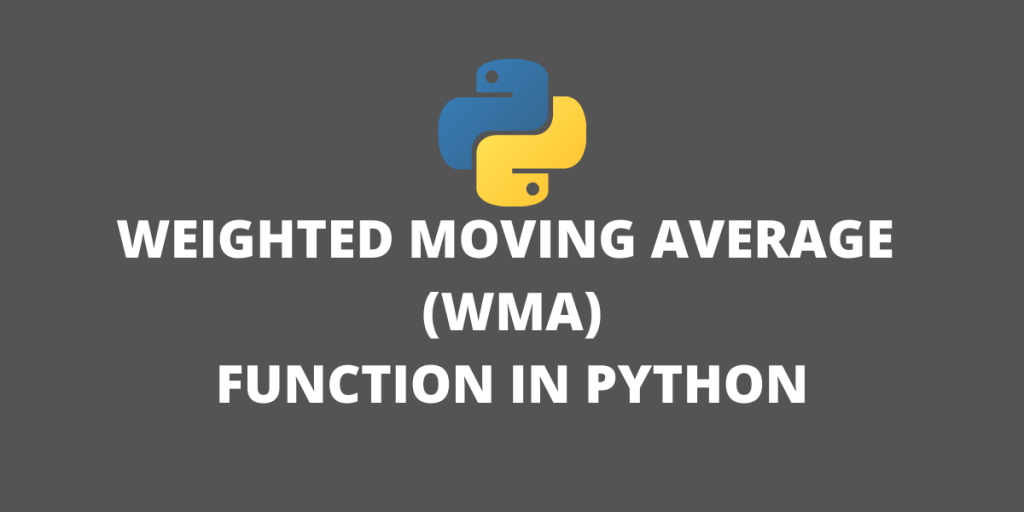# Weighted Moving Average – Implementation in PythonIn this article, we’ll calculate the Weighted Moving Average in Python. Weight Moving Average or WMA is used extensively in trading setups

## Understanding Moving Averages

A Moving Average is used to analyze the time-series data by calculating averages of different subsets of the complete dataset. Moving Average is also known as Rolling or Running Average.

It is also known as Rolling Mean or Moving Mean because it includes taking the average of the dataset. Moving average is mostly used with time-series data to capture the short-term fluctuations while focusing on longer trends. It smoothens the data.

ARIMA(Autoregressive Integrated Moving Average Model) algorithm uses moving averages to make time-series data predictions.

Examples: Stock prices, weather reports, air quality, gross domestic product, employment, etc.

## Types of Moving Averages

• Simple Moving Averages (SMA)
• Cumulative Moving Averages (CMA)
• Exponential Moving Averages (EMA)
• Weighted Moving Average (WMA)

## Simple Moving Average (SMA)

Simple Moving Average (SMA) makes use of the sliding window to take the average over a set number of time periods. The Simple Moving Average is only one of several moving averages available that can be applied to price series to build trading systems or investment decision frameworks. Among those, two other moving averages are commonly used among financial market are:

• Weighted Moving Average
• Exponential Moving Average

## Weighted Moving Average (WMA)

The weighted moving average (WMA) is a technical indicator that assigns a greater weighting to the most recent data points, and less weighting to data points in the distant past.

We obtain WMA by multiplying each number in the data set by a predetermined weight and summing up the resulting values. WMA is used by traders to generate trade signals, to indicate when to purchase or sell stocks.

Here’s a simple example:

Suppose you need to calculate the WMA of 3 closing prices on the daily chart. The prices are ₹10, ₹12, ₹15 respectively where ₹15 is the latest price.

A weight is assigned to each price based on the recency of the price. So in this case, ₹15 will be assigned a weight of 3, ₹12 will get a weight as 2, and ₹10 will get a weight as 1. Remember, this is an oversimplified calculation and in real-world scenarios, the calculations can have weights as decimal points too.

Next, we’ll calculating the sum of the weights of the time period so 1 + 2 + 3 = 6.

Finally, we’ll compute the WMA with the weights as follows:

[(₹15 * 3) + (₹12 * 2) + (₹10 * 1)]/6 = 13.1666666667

In our calculation, the 3-period WMA of the above prices is 13.1666666667.

## Implementing the Weighted Moving Average Formula in Python

Let’s not work with implementing the WMA formula we talked about earlier, in Python. The below function can be used on any time-series data that you pass to the function.

```def weightedmovingaverage(Data, period):
weighted = []
for i in range(len(Data)):
try:
total = np.arange(1, period + 1, 1) # weight matrix
matrix = Data[i - period + 1: i + 1, 3:4]
matrix = np.ndarray.flatten(matrix)
matrix = total * matrix # multiplication
wma = (matrix.sum()) / (total.sum()) # WMA
weighted = np.append(weighted, wma) # add to array
except ValueError:
pass
return weighted
```

Output with randomized pricing data:

## Conclusion

Weighted moving averages assign a heavier weighting to more current data points since they are more relevant than data points in the distant past. The sum of the weighting should add up to 1 (or 100 percent). In the case of the simple moving average, the weightings are equally distributed.

Stay tuned for more articles on Python!